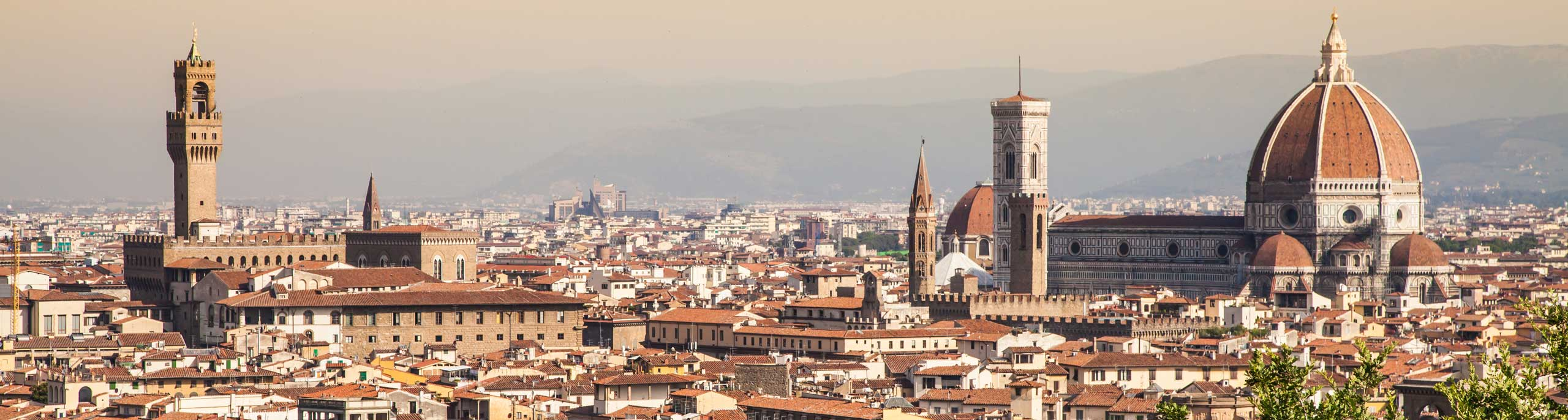The product of two positive consecutive integers is equal to 56. Find the two integers. The sum of the squares of two consecutive numbers is equal to 145. Find the two numbers. A rectangular garden has length of x + 2 and width of x + 1 and an area of 42. Find the perimeter of this garden A right triangle has one leg 3 cm longer that the other leg. Its hypotenuse is 3 cm longer than its longer leg. What is the length of the hypotenuse? The height h above the ground of an object propelled vertically is given by h = -16 t2 + 64 t + 32 , where h is in feet and t is in seconds. At what time t will the object be 80 feet above ground? The area of a rectangle is equal to 96 square meters. Find the length and width of the rectangle if its perimeter is equal to 40 meters. The height of a triangle is 3 feet longer than its corresponding base. The area of the triangle is equal to 54 square feet. Find the base and the height of the triangle. The product of the first and the third of three consecutive positive integers is equal to 1 subtracted from the square of the second of these numbers. Find the three integers. The product of two positive numbers is equal to 2 and their difference is equal to 7/2. Find the two numbers. The sum of the squares of three consecutive integers is equal to 77. What are the three integers?